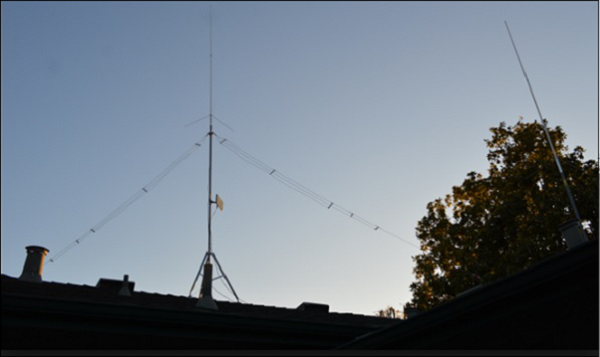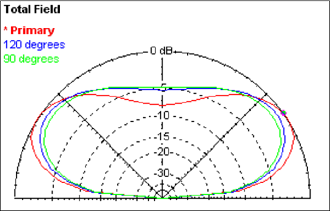# Antenna Theory - Inverted V-Antenna

In the previous chapter, we have studied V-antenna. Its operating frequency is limited. This can be modified by using another antenna, which is a non-resonant antenna or a travelling wave antenna. A travelling wave antenna produces no standing wave, as discussed previously.

### Frequency Range

The frequency range of operation of an inverted vee antenna (or V-antenna) is around 3 to 30 MHz. This antenna works in high frequency range.

## Construction & Working of Inverted V-Antenna

A travelling wave antenna, used in high-frequency band is an inverted V-antenna. This inverted V-antenna is easily installed on a non-conducting mast.

Take a look at the following image. It shows an inverted V-antenna mounted on a roof top.The maximum radiation for an inverted V-antenna is at its center. It is similar to a halfwave dipole antenna. The antenna is placed in the shape of an inverted V, with its two transmission lines or legs bent towards the ground making 120° or 90° angle between them. The center of the antenna should not be higher than λ/4.

The angle made by one of the legs with the axis of the antenna, is known as the tilt angle and is denoted by θ.

The radiation pattern of inverted V-antenna is uni-directional pattern, as no standing waves are formed here. It can be clearly understood by the radiation pattern shown below.The figure illustrates the radiation pattern of an inverted V-antenna. Primary radiated field is shown along with the fields when the tilt angles are 120˚ and 90˚ in the figure given above. The gain and directivity are improved by having an array of antennas.

The following are the advantages of inverted V-antenna −

• Occupies less horizontal place

• No standing waves are formed

• High gain

The following are the disadvantages of inverted V-antenna −

• It has considerable undesired minor lobes

• Minor lobes create horizontally polarized waves

### Applications

The following are the applications of inverted V-antenna −

• Used in tuned circuit applications